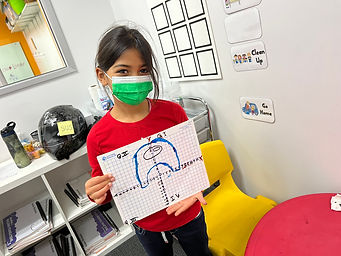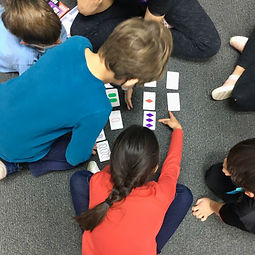## Ms. AJ

### Target 1​

###### Lesson Type:

Continuation

Geometry

:

Coordinates

Graph shapes in the first two quadrants of the coordinate plane.

###### 1:

Understand that geometric figures can be drawn on the coordinate plane.

###### 2:

Become familiar with how the coordinate plane can be used to determine distance and length of geometric shapes.

5th

###### Vocabulary:

Coordinate, Coordinate Pair, Quadrants I II III & IV, Plot, X-Axis, Y-Axis, Negative Numbers

Activities:

Students set up their coordinate grids, utilizing all four quadrants, labeling everything accordingly.

Students plotted given coordinates to create a figure on their coordinate grids, and took note of the reflection of certain points over the y-axis and how the coordinates compared.### Home Exploration

###### Guiding Questions:## Absent Students:

### Target 2

:

###### 1:

Identify the x- and y-axis on a coordinate plane.

###### 2:

Become familiar with quadrant I of the coordinate plane and that all coordinate pairs will have positive numbers in this quadrant.

###### 3:

Become familiar with quadrant II of the coordinate plane and that the first number can be either positive or negative.

###### 4:

Understand that the first number in a coordinate pair indicates placement on the x-axis and the second indicates placement on the y-axis.

###### 5:

Graph points in the first and second quadrant of the coordinate plane.

5th

###### Vocabulary:

Coordinate, Coordinate Pair, Quadrants I II III & IV, Plot, X-Axis, Y-Axis, Negative Numbers

Activities:

Students played "Battleship", utilizing all four quadrant spaces o practice locating coordinates on their coordinate grids.### Home Exploration

###### Guiding Questions:### Target 3

:

###### 1:

Use the given information in a problem to identify the correct answer.

###### 2:

Use the given information to determine which is not the correct answer.

###### 3:

Think outside of the box to solve problems.

###### 4:

Follow given rules to solve problems.

3rd

###### Vocabulary:

Attribute

Activities:

Students were introduced to the game "SET", in which they were to use process of elimination to create sets of three cards with the right kinds and numbers of attributes.### Home Exploration